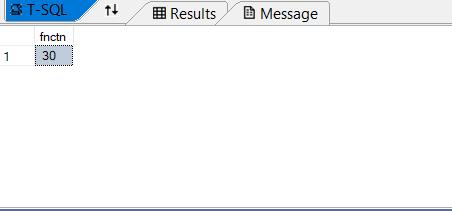Loading, please wait...# How to call a SQL function with a Stored Procedure?

In this article , we will talk about how we can call a function from a stored procedure in SQL.

There are two types of functions in SQL Server. These are:

• System defined functions
• User-defined functions

Again, user-defined functions are also further classified into three types:

1. Scalar function: These functions return a single value as a result of tasks executed by the function.
2. Inline table-valued functions: A result in the form of a table is returned by these functions on execution.
3. Multi-statement Table-valued functions: user-defined multi-statement table-valued function values are generated as a result of these functions being executed.

Here, we will show how we can call a function with the help of a stored procedure in SQL Server with the help of a simple example.

Let's take a scalar function named Fnctn which will take two integer values and return their product in a third integer value.

### Creating a user-defined scalar function in SQL Server:

let us quickly create a function named Fnctn with two parameters num1 and num2 returning a result in another parameter named ‘res’.

Both the parameters are of the same data type .i.e. int.

The function is given below:

``````create function [dbo].[fnctn]
(
@num1 int,
@num2 int
)
returns int
as
begin

declare @res int
select @res = @num1 * @num2;
return @res

end``````

### creating a stored procedure in SQL Server

we can call a function using a select command or by using it in a stored procedure.

The function would return a value as a result, therefore we need a parameter in the stored procedure as well so as to hold the result value in it.

A stored procedure including a function in it is given below for an instance.

``````create procedure [dbo].[SP1]
(
@p1 int, @p2 int
)
as
begin

declare @pro int
select [dbo].[fnctn](@p1 , @p2)

end``````

we can call the function as :

``select dbo.[fnctn](3,10) as fnctn``

The result will be like:Explanation:

the values 3 and 10 are passed in the two parameters which generate a result as 30 by using the function with a stored procedure.